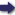## Bīrūnī, Muḥammad ibn Aḥmad, Alberuni's India (v. 2)

(London :  Kegan Paul, Trench, Trübner & Co.,  1910.)

 Tools

## Search this bookPrev Page 207 Next``` CHAPTER LXXIX. 207 kept in memory. If the corrected place of the moon Another is less than three zodiacal signs, it is that which we the author want; if it is between three and six signs, he subtracts natnaka. it from six signs, and if it is between six and nine signs, he adds six signs thereto ; if it is more than nine signs, he subtracts it from twelve signs. Thereby he gets the second place of the moon, and this he compares with the moon's place at the time of the correction. If the second place of the moon is less than the first, the time of the two declinations equalling each other is future; if it is more than the first, the time of their equalling each other is past. Further, he multiplies the difference between the two places of the moon by the bhukti of the sun, and divides the product by the bhukti of the moon. The quotient he adds to the place of the sun at the time of the cor¬ rection, if the second place of the moon is larger than the first; but he subtracts it from the sun's place, if the second place of the moon is less than the first. Thereby he finds the place of the sun for the time when the two declinations are equal to each other. For the purpose of finding it, he divides the difference between the two places of the moon by the bhukti of the moon. The quotient gives minutes of days, indi¬ cative of the distance. By means of them he com¬ putes the places of sun and moon, of the dragon's head and tail, and of the two declinations. If the latter are equal, it is that which we want to find. If they are not equal, the author repeats the calculation so long till they are equal and till the correct time has been found. Thereupon he computes the measure of sun and moon. However, he disregards half of the sum of them, so that in the further calculation he uses only the one half of their measures. He multiplies it by 60 and divides the product by the bhuktyantarct. The quotient represents the minutes of the falling (pdtct ?). ```Prev Page 207 Next